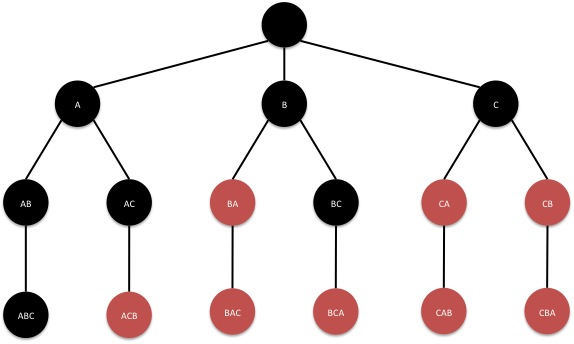# DFS in Binary TreeLet’s think about this problem: Sum Root to Leaf Numbers.

A general solution is like below:

Actually the core part for DFS recursion is the last two calls of helper() method:

In this way, we have traverse the tree in pre-order. So this is the essence of DFS for binary trees. Then we just include three other pieces, and we are done with this sort of questions.

1. Checking null for the current node is a routine operation. This means we have reached the bottom of this iteration, either on the left side or the right side, then we return to the previous level of the stack.
2. Do something about the current node. This is the actually what the problem is asking for. In the example above, we check if we have reached the leaf node. If we do, add the update the preliminary result into the ArrayList. If we need to use backtrack to record the results, don’t forget to remove the last added element from the preliminary result before we continue.
3. The order of the recursion depends on whether it is pre-order, in-order, or post-order.

A similar problem is: Path Sum II